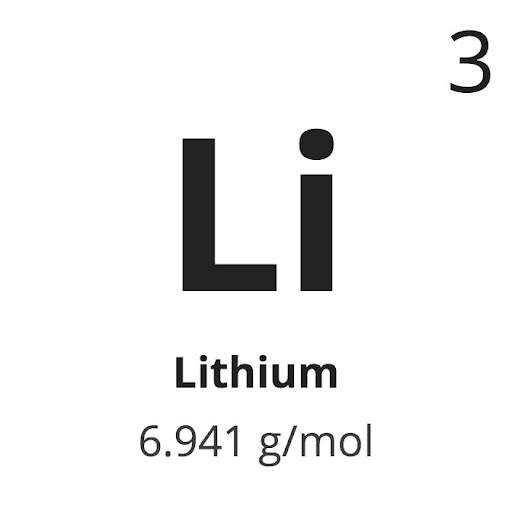# Generalized Ito formula for the functions in Sobolev functions

The generalized Ito formula to the Sobolev spaces will be discussed here, which stems from [Kry80]. I would like to thank Prof Krylov on his confirmation of this result by email communication.

Proposition 1 Let${X}$ be a diffusion on the filtered probability space${(\Omega, \mathcal{F}, \mathbb{P}, \mathbb{F}= \{\mathcal{F}_t\})}$, with generator${L}$, initial time${s}$, and initial state${x\in \mathbb{R}^d}$. Suppose${v\in W_{d+1,loc}^{1,2}(Q)}$ for some${Q\subset \mathbb{R}^{d+1}}$. Define$\displaystyle \tau_Q^{s,x} = \inf\{r>s: X_r \notin Q\}.$

Then, for any${\mathbb{F}}$-stopping time${\tau \le \tau_Q^{s,x}}$, we have$\displaystyle \mathbb{E}[v(\tau, X_\tau)] = v(s,x) + \mathbb{E} \Big[ \int_s^\tau L v(r,X_r) dr\Big]. \ \ \ \ \ (1)$

Proof:

For any${\mathbb{F}}$-stopping time${\tau \le \tau_Q^{s,x}}$, we have inequality, by Theorem 2.10.2 of book [Kry80],$\displaystyle \mathbb{E}[v(\tau, X_\tau)] \le v(s,x) + \mathbb{E} \Big[ \int_s^\tau L v(r,X_r) dr\Big]. \ \ \ \ \ (2)$

If we apply (2) to a function${v_1 = -v}$, then$\displaystyle \mathbb{E}[v_1(\tau, X_\tau)] \le v_1(s,x) + \mathbb{E} \Big[ \int_s^\tau L v_1(r,X_r) dr\Big],$

which yields$\displaystyle \mathbb{E}[v(\tau, X_\tau)] \ge v(s,x) + \mathbb{E} \Big[ \int_s^\tau L v(r,X_r) dr\Big]. \ \ \ \ \ (3)$

From (2) and (3), we conclude equality holds for (2).$\Box$

## One comment

1.Joe Li says:|

Nice. Do you know any reference on this generalized Dynkin’s Formula? I’m curious what Prof. Krylov responded.